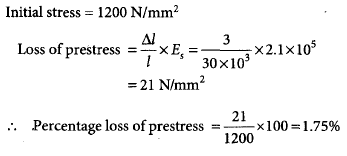Courses

# Test: RCC (Concrete Structures)- 1

## 15 Questions MCQ Test GATE Civil Engineering (CE) 2022 Mock Test Series | Test: RCC (Concrete Structures)- 1

Description
This mock test of Test: RCC (Concrete Structures)- 1 for GATE helps you for every GATE entrance exam. This contains 15 Multiple Choice Questions for GATE Test: RCC (Concrete Structures)- 1 (mcq) to study with solutions a complete question bank. The solved questions answers in this Test: RCC (Concrete Structures)- 1 quiz give you a good mix of easy questions and tough questions. GATE students definitely take this Test: RCC (Concrete Structures)- 1 exercise for a better result in the exam. You can find other Test: RCC (Concrete Structures)- 1 extra questions, long questions & short questions for GATE on EduRev as well by searching above.
QUESTION: 1

### The lap length of reinforcement in compression should not be less than

Solution: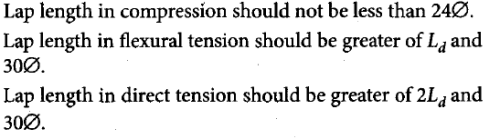QUESTION: 2

### A reinforced concrete slab is 75 mm thick. The maximum size of reinforcement bar that can be used is

Solution:

Maximum size of bar that can be used as an reinforcement is less than (total depth)/8= 75/8 =9.375mm
Therefore, from options max. size that can be used is 8mm.

QUESTION: 3

### A river 5 m deep consists of a sand bed with saturated unit weight of 20 kN/m3 . vw = 9.81 kN/m3. The effective vertical stress at 5 m from the top of sand bed is

Solution: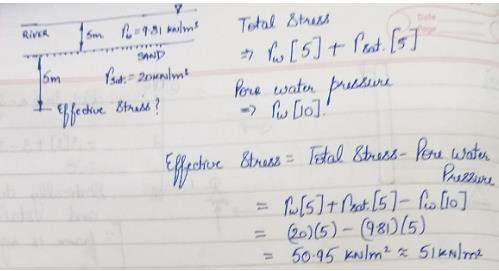QUESTION: 4

For the design of a simply supported T-beam the ratio of the effective span to the overall depth of the beam is limited to

Solution:

BASIC SPAN to EFFECTIVE DEPTH RATIO
Cantilever    7
Simply Supported Beam    20
Continuous Beam    26

QUESTION: 5

In the design of two-way slab restrained at all edges, torsional reinforcement required is:

Solution:

TORSION REINFORCEMENT
0.75 Ast max where both edges are discontinuous (restrained at all edges)
(0.75/2) Ast max if only one edge discontinuous
No torsion reinforcement where both edges are discontinuous
Where Ast max is the maximum tension reinforcement provided in the slab (usually at midspan)

QUESTION: 6

A prestressed rectangular beam which carries two concentrated loads W at L/3 from either end, is provided with a bent tendon with tension P such that central one-third portion of the tendon remains parallel to the longitudinal axis, the maximum dip h is

Solution:
QUESTION: 7

In the prestressed concrete beam section shown in the given figure (all dimensions in mm in the figure), if the net losses are 15% and the final prestressing force applied at A is 500 kN, the initial extreme fiber stresses at the top and bottom will be, respectively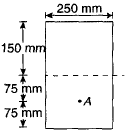Solution: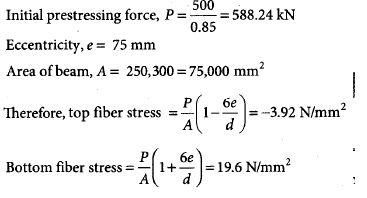QUESTION: 8

A simply supported rectangular beam of span 20.0 m is subjected to uniformly distributed load (UDL). The minimum effective depth required to check the deflection of this depth, when modification factors for tension and compression are 0.9 and 1.1, respectively, will be _________ m.

Solution: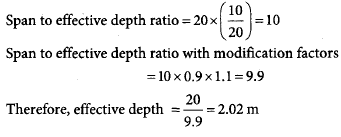QUESTION: 9

The permissible bending stresses Pbet for steel slab bases should not exceed

Solution: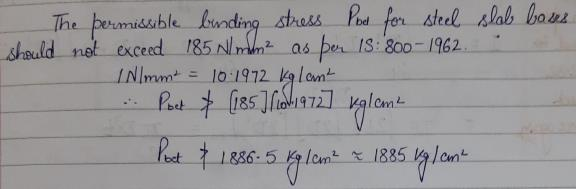QUESTION: 10

An ordinary mild steel bar has been prestressed to a working stress of 200 MPa. Young’s modulus is 200 GPa. Permanent negative strain due to shrinkage and creep is 0.0008. How much is the effective stress left in steel?

Solution: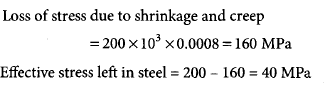QUESTION: 11

What is the net downward load to be considered for the analysis of the prestress concrete beam provided with a parabolic cable as shown in the figure below?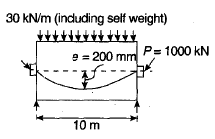Solution: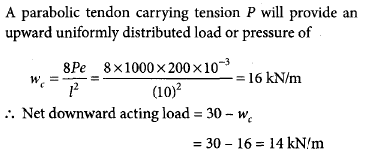QUESTION: 12

What should be the maximum area of reinforcement (i) in compression and (ii) in tension to be provided in an RC beam, respectively, as per

Solution: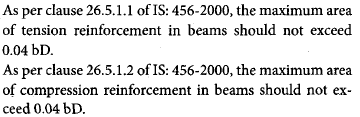QUESTION: 13

If a simply supported concrete beam, prestressed with a force of 2500 kN is designed by load balancing concept for an effective span of 10 m and to carry a total load of 40 kN/m, the central dip of the cable profile should be?

Solution: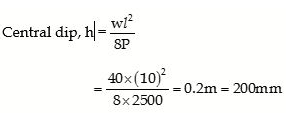QUESTION: 14

The development length in compression for a 20 mm diameter deformed bar of grade Fe415 embedded in concrete of grade M25 whose design bond stress is 1.40 N/mm2 is

Solution: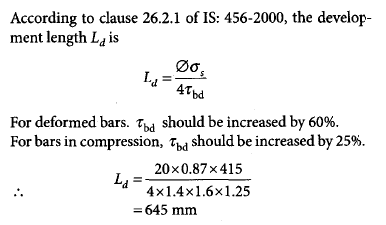QUESTION: 15

The percentage loss of prestress due to anchorage slip of 3 mm in a concrete beam of length 30 m which is post-tensioned by a tendon with an initial stress of 1200 N/mm2 and modulus of elasticity equal to 2.1 × 105 N/mm2 is

Solution: